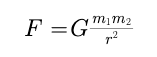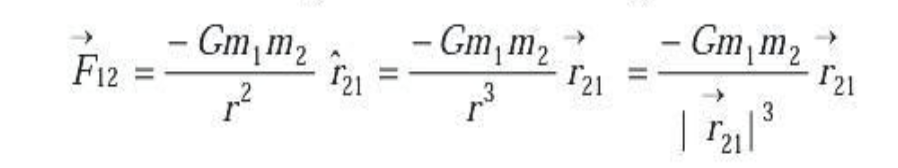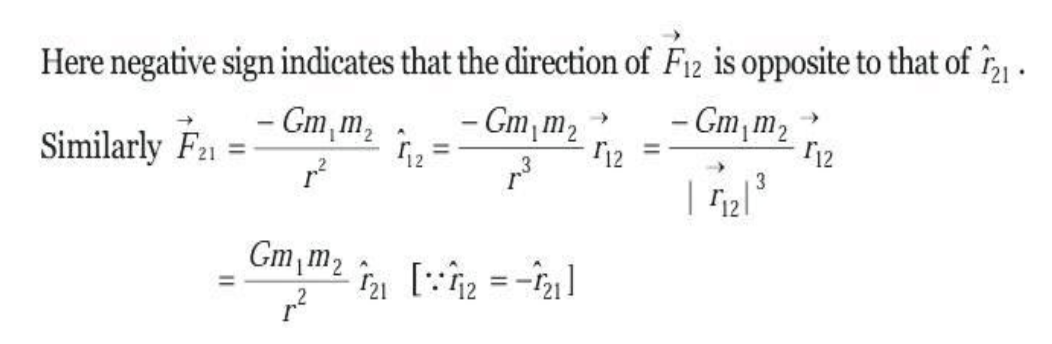# Law Of Gravitation. - Gravitation

Law Of Gravitation

Introduction

Newton at the age of twenty-three is said to have seen an apple falling down from tree in his orchid. This was the year 1665. He started thinking about the role of earth's attraction in the motion of moon and other heavenly bodies.  By comparing the acceleration due to gravity due to earth with the acceleration required to keep the moon in its orbit around the earth, he was able to arrive the Basic Law of Gravitation.

Newton Law Of Gravitation:

Newton's law of gravitation states that every orVector Form : According to Newton's law of gravitationbody in this universe attracts every other body with a force, which is directly proportional to the product of their masses and inversely proportional to the square of the distance between their centres. The direction of the force is along the line joining the particles.  Thus the magnitude of the gravitational force F that two particles of masses m1 and m2 separated by a distance r exert on each other is given by